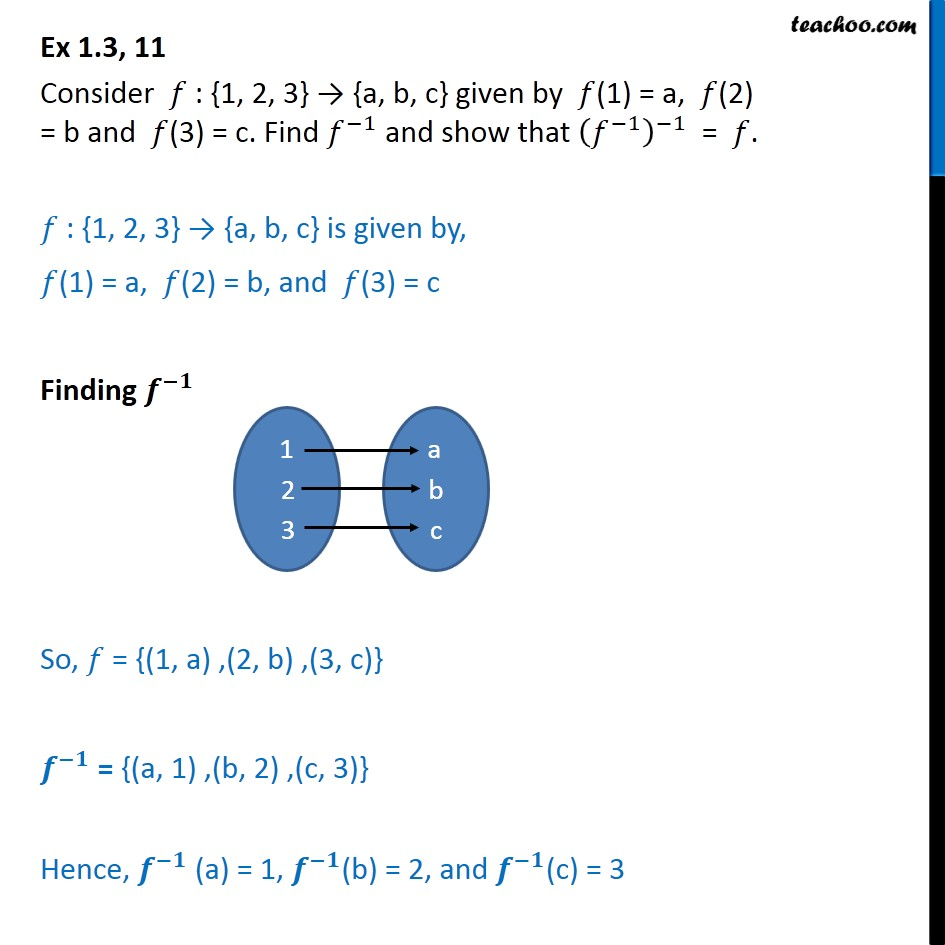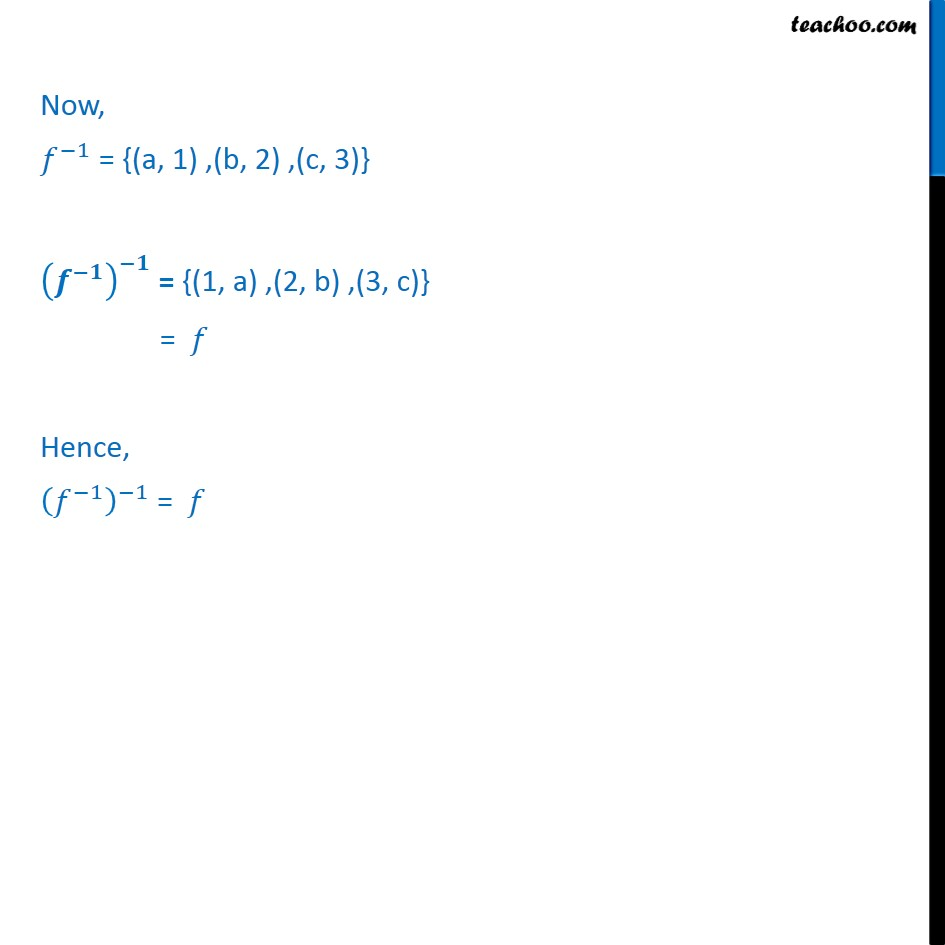Finding Inverse

Chapter 1 Class 12 Relation and Functions
Concept wiseLearn in your speed, with individual attention - Teachoo Maths 1-on-1 Class

### Transcript

Ex 1.3, 11 Consider : {1, 2, 3} {a, b, c} given by (1) = a, (2) = b and (3) = c. Find 1 and show that 1 1 = . : {1, 2, 3} {a, b, c} is given by, (1) = a, (2) = b, and (3) = c Finding So, = {(1, a) ,(2, b) ,(3, c)} = {(a, 1) ,(b, 2) ,(c, 3)} Hence, (a) = 1, (b) = 2, and (c) = 3 Now, 1 = {(a, 1) ,(b, 2) ,(c, 3)} = {(1, a) ,(2, b) ,(3, c)} = Hence, 1 1 =